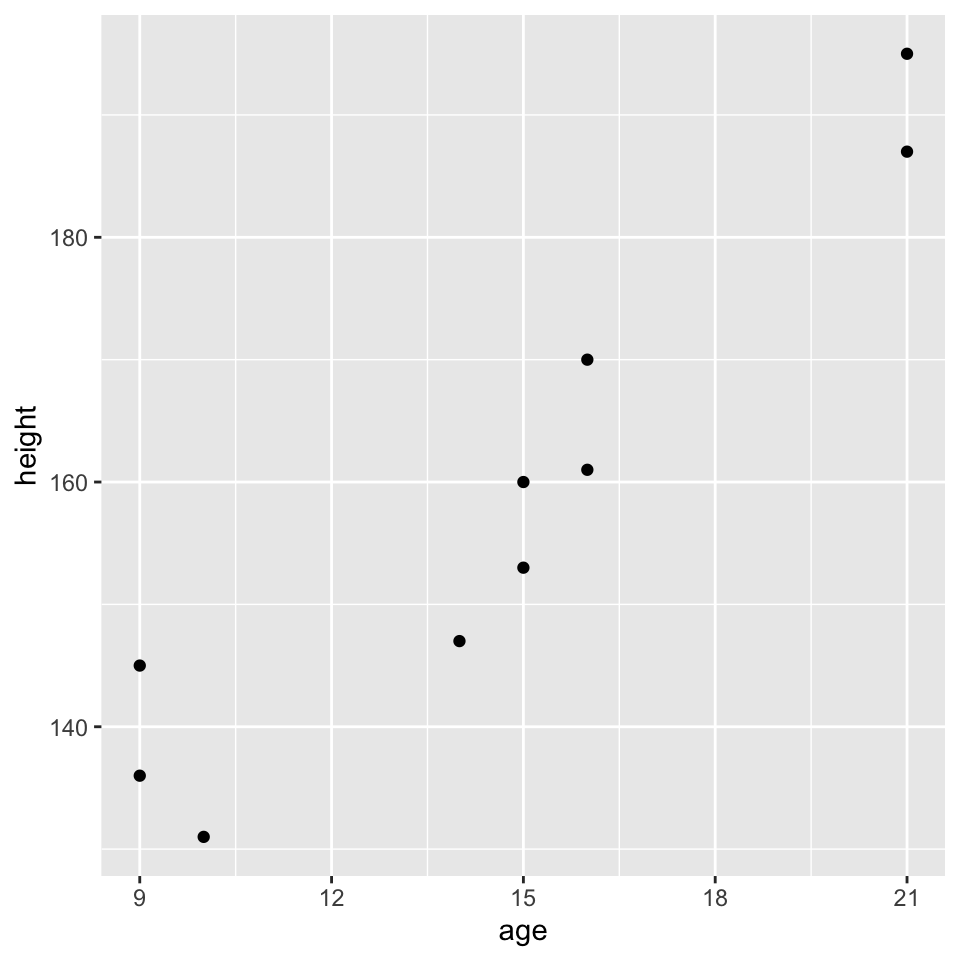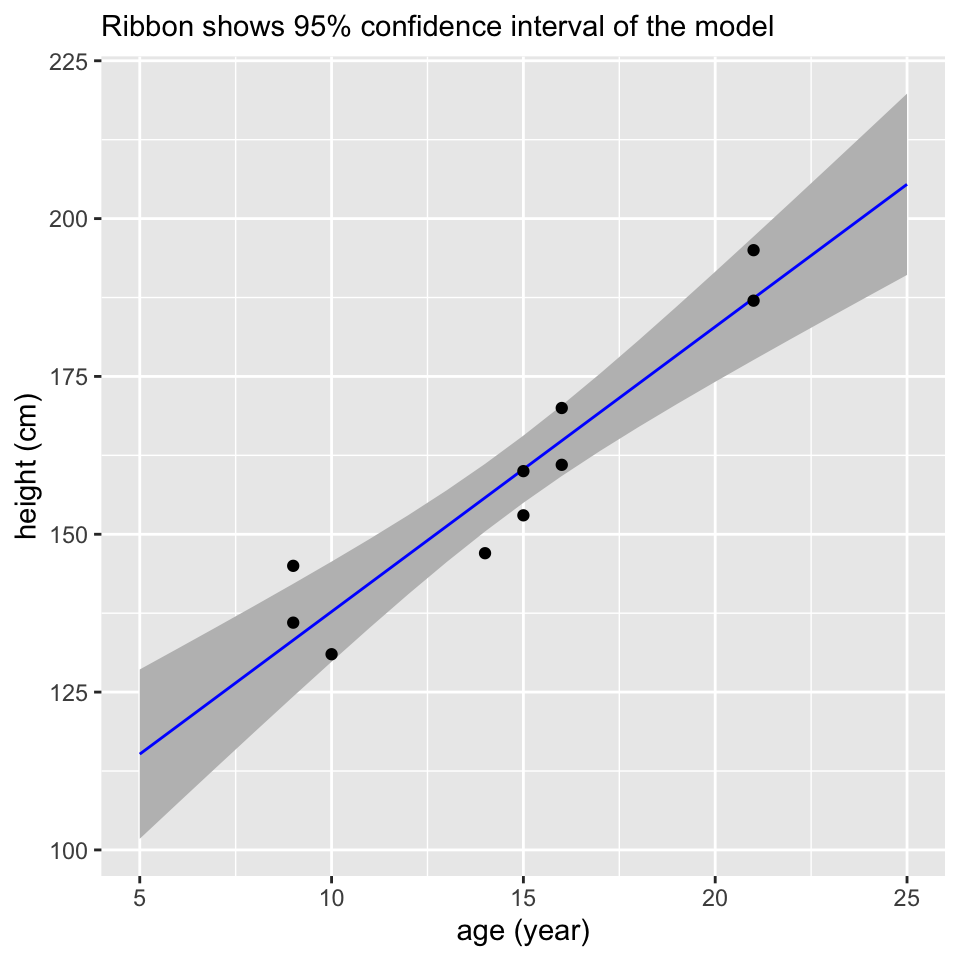``````library(MASS)       # ginv -- coefficient estimation
library(splines)    # ns, bs -- spline curves
library(multcomp)   # glht -- linear hypotheses
library(edgeR)      # cpm, etc -- RNA-Seq normalization
library(limma)      # lmFit, etc -- fitting many models
library(tidyverse)  # working with data frames, plotting``````

Much of what we will be using is built into R without loading any packages.

# 2 Vectors and matrices

## 2.1 Vector operations

``````a <- c(3,4)
b <- c(5,6)

length(a)``````
``##  2``

R performs operations elementwise:

``a + b``
``##   8 10``
``a * b``
``##  15 24``

We will be using the dot product a lot. This is:

``sum(a*b)``
``##  39``
``t(a) %*% b``
``````##      [,1]
## [1,]   39``````

The geometric length of a vector is (by Pythagorus):

``sqrt(sum(a*a))``
``##  5``

## 2.2 Matrix operations

We can create a matrix with `matrix`, `rbind` (row bind), or `cbind` (column bind).

``matrix(c(1,2,3,4), nrow=2, ncol=2)``
``````##      [,1] [,2]
## [1,]    1    3
## [2,]    2    4``````
``rbind(c(1,3), c(2,4))``
``````##      [,1] [,2]
## [1,]    1    3
## [2,]    2    4``````
``cbind(c(1,2), c(3,4))``
``````##      [,1] [,2]
## [1,]    1    3
## [2,]    2    4``````
``````X <- rbind(
c(1,0),
c(1,0),
c(1,1),
c(1,1))
X``````
``````##      [,1] [,2]
## [1,]    1    0
## [2,]    1    0
## [3,]    1    1
## [4,]    1    1``````
``class(X)``
``##  "matrix"``

The matrix transpose is obtained with `t`.

``t(X)``
``````##      [,1] [,2] [,3] [,4]
## [1,]    1    1    1    1
## [2,]    0    0    1    1``````

Matrix multiplication is performed with `%*%`. The dot product of each row of the left hand side matrix and each column of the right hand side matrix is calculated. `%*%` treats a vector as either a single column or single row matrix as will make sense for matrix multiplication. Actually all we need today is to multiply a matrix by a vector, in which case we get the dot product of each row of the matrix with the vector.

``X %*% a``
``````##      [,1]
## [1,]    3
## [2,]    3
## [3,]    7
## [4,]    7``````
``as.vector(X %*% a)``
``##  3 3 7 7``

## 2.3 Challenge - use a dot product to calculate

The following dot product is an elaborate way to retrieve `x`:

``````x <- c(10,20,30,40)
weights <- c(0,1,0,0)       # <-- modify this line
sum(weights*x)``````

Modify `weights` in the above to calculate different quantities:

A. `x-x`

B. The mean of all four values.

# 3 Single numerical predictor

The age (year) and height (cm) of 10 people has been measured. We want a model that can predict height based on age.

``````people <- read_csv(
"age, height
10,    131
14,    147
16,    161
9,    136
16,    170
15,    160
15,    153
21,    187
9,    145
21,    195")

ggplot(people, aes(x=age, y=height)) + geom_point()````````````fit <- lm(height ~ age, data=people)

fit``````
``````##
## Call:
## lm(formula = height ~ age, data = people)
##
## Coefficients:
## (Intercept)          age
##      92.612        4.513``````

Coefficients are extracted with `coef`:

``coef(fit)``
``````## (Intercept)         age
##   92.611502    4.512911``````

The residual standard deviation is extracted with `sigma`:

``sigma(fit)``
``##  7.263536``

Behind the scenes a matrix of predictors has been produced from the mysterious notation `~ age`. We can examine it explicitly:

``model.matrix(fit)``

`model.matrix` can be used without first calling `lm`.

``model.matrix(~ age, data=people)``
``````##    (Intercept) age
## 1            1  10
## 2            1  14
## 3            1  16
## 4            1   9
## 5            1  16
## 6            1  15
## 7            1  15
## 8            1  21
## 9            1   9
## 10           1  21
## attr(,"assign")
##  0 1``````

n=10 observations minus p=2 columns in the model matrix leaves 8 residual degrees of freedom:

``df.residual(fit)``
``##  8``

## 3.1 Prediction

`predict` predicts. By default it produces predictions on the original dataset.

``predict(fit)``
``````##        1        2        3        4        5        6        7        8
## 137.7406 155.7923 164.8181 133.2277 164.8181 160.3052 160.3052 187.3826
##        9       10
## 133.2277 187.3826``````
``predict(fit, interval="confidence")``
``````##         fit      lwr      upr
## 1  137.7406 129.8100 145.6712
## 2  155.7923 150.4399 161.1446
## 3  164.8181 159.2250 170.4111
## 4  133.2277 124.3009 142.1545
## 5  164.8181 159.2250 170.4111
## 6  160.3052 154.9836 165.6267
## 7  160.3052 154.9836 165.6267
## 8  187.3826 177.6105 197.1547
## 9  133.2277 124.3009 142.1545
## 10 187.3826 177.6105 197.1547``````

We can also calculate predictions manually.

``````# Prediction for a 15-year old
x <- c(1, 15)
beta <- coef(fit)
sum(x * beta)``````
``##  160.3052``
``````# Prediction for all original data
X <- model.matrix(fit)
as.vector( X %*% beta )``````
``````##   137.7406 155.7923 164.8181 133.2277 164.8181 160.3052 160.3052 187.3826
##   133.2277 187.3826``````

`predict` can be used with new data.

``````new_people <- tibble(age=5:25)
predict(fit, new_people)``````
``````##        1        2        3        4        5        6        7        8
## 115.1761 119.6890 124.2019 128.7148 133.2277 137.7406 142.2535 146.7664
##        9       10       11       12       13       14       15       16
## 151.2793 155.7923 160.3052 164.8181 169.3310 173.8439 178.3568 182.8697
##       17       18       19       20       21
## 187.3826 191.8955 196.4085 200.9214 205.4343``````
``````new_predictions <- cbind(
new_people,
predict(fit, new_people, interval="confidence"))

ggplot() +
geom_ribbon(aes(x=age, ymin=lwr, ymax=upr), data=new_predictions, fill="grey") +
geom_line(aes(x=age, y=fit), data=new_predictions, color="blue") +
geom_point(aes(x=age, y=height), data=people) +
labs(y="height (cm)", x="age (year)",
subtitle="Ribbon shows 95% confidence interval of the model")``````If you have ever used `geom_smooth`, it should now be a little less mysterious.

``ggplot(people, aes(x=age, y=height)) + geom_smooth(method="lm") + geom_point()``